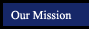Geometry Postulates and Theorems
textbook tutorials - definitions - constructions - postulates and theorems - internet activities and resources
 symmetry and similarity points, lines and planes parallel lines triangles quadrilaterals polygons circles 3-Dimensional Geometry
Volume
Surface Area
Graphing in 3 Dimensions
The volume of a pyramid is one third the product of the height and the area of the base (V = 1/3 Bh). The surface area of the regular pyramid is the sum of the lateral area and the area of the base (S.A. = 1/2ps + B) The midpoint of the segment that joins any two points A(x1,y1,z1) and B(x2,y2,z2) has the coordinates[(x1 + x2)/2, [(y1 + y2)/2,[(z1 + z2)/2].
The volume of a cone is one third the product of the height and the area of the base (V = 1/3 (pi)r2h)  The surface area of a cone is the sum of the lateral area and the area of the base (S.A. = (pi)rs + (pi)r2). The distance AB between two points in space A(x1,y1,z1) and B(x2,y2,z2) is square root of (x2 - x1)2 + (y2-y1)2 + (z2 + z1)2.
The volume of a sphere with radius r is 4/3(pi)r3(V = 4/3 (pi)r3. The surface areaa of a sphere with radius r is 4(pi)r2. (S.A. = 4(pi)r^2.